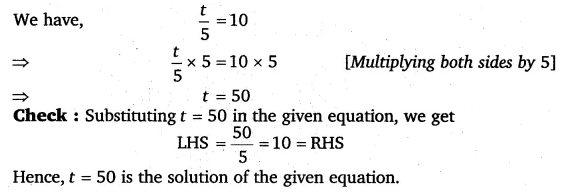# Solve the following equations: t/5 = 10

Solve the following equations: